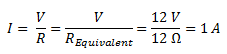# Resistors in Series and Parallel Combinations

## Resistors in Series and Parallel Combinations

In our previous post about resistors , we studied about different types of resistors.

In some cases when we do not get the desires or specific resistor values we have to either use variable resistors such as potentiometers or presets to obtain such precise values. However,  such pots are too expensive to use for every case.

Another method to do this,  is to combine two or more resistors to obtain the necessary precise values.Such resistor combinations cost very less.

Now the question arises as to how one should combine these resistors.

The resistors  can be combined in two different ways such as :

1. Series Combinations
2. Parallel combinations

### Resistors in Series

Resistors are said to be connected in “Series“, when they are daisy chained together in a single line.

Calculating values for two or more resistors in series is simple, just add all the values up.

The  series connection ensures that the SAME current flows through all resistors.

In this type of connection R Total  will always be GREATER than any of the included resistors.

The total resistance is the sum of all the resistors connected in series and is given by the expression  :

R Total = R1 + R2 + R3 +…………

#### Example :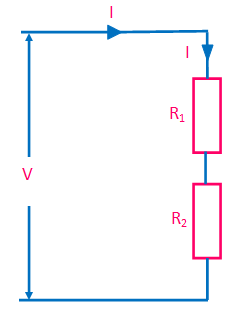• As the resistors are connected together in series,  the same current passes through each resistor in the chain and the total resistance, RTotal of the circuit must be equal to the sum of all the individual resistors added together. That is

R Total = R1 + R2

• The total applied voltage  V is divided by the two resistors.
• The current in the circuit is given as:
•  Using Ohm’s Law,the voltages across R1 and R2 are  given as :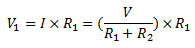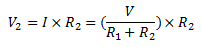• Hence, the total voltage is given as :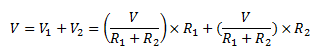• For example if we take V= 6 V , R1= 1 kΩ  and  R= 2 kΩ, then

R Total = 1 kΩ+ 2 kΩ = 3 kΩ

I = 6V/3kΩ = 2 mA

Voltage across the 1 kΩ resistor is      V1 =  2 mA × 1 kΩ = 2 V

Voltage across the 2 kΩ resistor is      V2 =  2 mA × 2 kΩ = 4 V

So we see that we can replace the two individual resistors above with just one single “equivalent” resistor                     which will have a value of 3 .

This total resistance is generally known as the Equivalent Resistance and can be defined as;  “a single value of resistance that can replace any number of resistors in series without altering the values of the current or the voltage in the circuit“.

The series connection can be  characterized by the following points :

1. The same current flows through all the resistors connected in series.
2. The resultant resistor is the SUM of all resistors in series.
3. Series resistors divide the total applied voltage proportional to their magnitude.

### Voltage Divider Circuit

Since the series resistors divide voltage, this idea an be used to get smaller voltage from a power supply output.

For example, we have a power supply with 10V fixed output. But we want only 5V from it. How to get it ?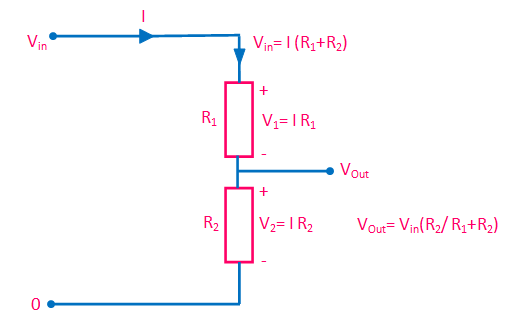The circuit shown above consists of  two resistors, R1 and R2 connected together in series across the supply voltage Vin.

The current I is given by: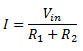Since the current I flows through   Ras well as R2, hence, by using Ohm’s law, the voltage developed across Ris given by :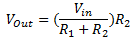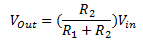If  R= R,    then Vout  = Vin  /2

If more resistors are connected in series to the circuit then different voltages will appear across each resistor in turn with regards to their individual resistance  values providing different but smaller voltage points from one single supply.

### Resistors in Parallel

Resistors are said to be connected together in “Parallel” when both of their terminals are respectively connected to each terminal of the other resistor or resistors.

### Parallel Combination

The fig. below shows the circuit of resistors in parallel combination where two resistors Rand R2  are connected in parallel across the supply voltage E .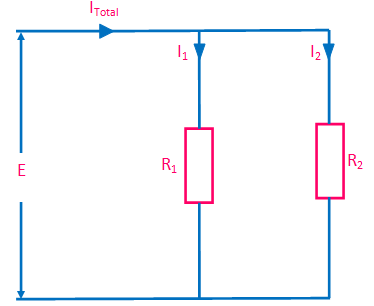As we can see from the fig. above :

• There are two paths available for Current. Hence current divides.
• But voltage across the resistors are the same.
•  If the two resistors are equal the current will divide equally and the RTotal will be exactly half of either resistor or exactly one third if there are three equal resistors.
• In general we can say :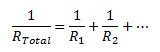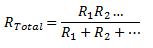### Currents in a Parallel Resistor Circuit

In a parallel resistor circuit the voltage remains same across each resistor connected in parallel. However,  the current through each parallel  resistor is not necessarily the same since the value of the resistance in each branch determines the current within that branch.

The total current, ITotal  in a parallel resistor circuit is the sum of the individual currents flowing in all the parallel branches which can be determined by using Ohm’s law.

#### ExampleLet’s take  the voltage E be 6V.

The resistors be R= 1 kΩ and R= 2 kΩ.

By using Ohm’s law , the current through R= 6 V/ 1 kΩ = 6 mA  and   current through R=  6 V/ 2 kΩ = 3 mA

Hence the total current is 6 mA + 3 mA = 9 mA

6 V will generate 9 mA only when the total resistance of the circuit is equal to :

6 V/ 9 mA = 0.66 kΩ

Hence the effective resistance of  Rand  Rconnected in parallel is 0.66 kΩ.

This effective resistance can also be calculated by using the  formula as below :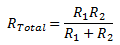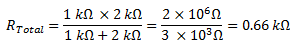Thus the parallel connection can be characterized by :

1. The same voltage exists across all the resistors connected in parallel .
2. The reciprocal of resultant or total resistance is the sum of reciprocals of all resistors in parallel .
3. Parallel resistors divide the total current in an inverse proportion to their magnitude.
4. When a set of resistors are connected in parallel, the effective resistance is always smaller then the smallest in the set.

For example:  Let 1 kΩ and 10 kΩ resistors are  in parallel .

Then the resultant is    (1 k × 10 k)/ 11 k = 0.9 kΩ , which is smaller than 1 k ( the smallest).

### Resistors in Series and Parallel Combinations

In some electrical and electronic circuits it is required to connect various resistors together in “BOTH” parallel and series combinations within the same circuit and produce more complex resistive networks.

Now the question arises, how do we calculate the combined or total circuit resistance, currents and voltages for these resistive combinations.

Resistor circuits that combine series and parallel resistors networks together are generally known as Resistor Combination or mixed resistor circuits.

The method of calculating the circuits equivalent resistance is the same as that for any individual series or parallel circuit.

The most important thing to keep in mind in such calculations is that resistors in series carry exactly the same current and that resistors in parallel have exactly the same voltage across them.

#### Example

Let us consider the circuit shown in fig. below :In the above circuit  let us calculate the total current ( IT ) taken from the 12 v supply.

We can see that the two resistors, R2 and R3 are actually connected in a “SERIES” combination so we can add them together to produce an equivalent resistance.  The resultant resistance for this combination would therefore be:

R2+ R= 8 Ω +4 Ω = 12 Ω

So we can replace both resistor R2 and R3 above with a single resistor of resistance value 12 Ω as shown in fig. below: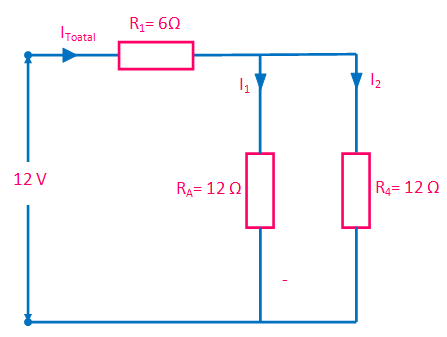So our circuit now has a single resistor RA in “PARALLEL” with the resistor R4. Using our resistors in parallel equation we can reduce this parallel combination to a single equivalent resistor value of R(combination) using the formula for two parallel connected resistors as follows.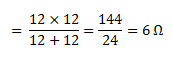The resultant resistive circuit now looks something like this:We can see that the two remaining resistances, R1 and R(combination) are connected together in a “SERIES” combination and again they can be added together (resistors in series) so that the total circuit resistance therefore given as: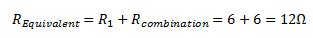A single resistance of just 12 Ω can be used to replace the original four resistors connected together in the original circuit.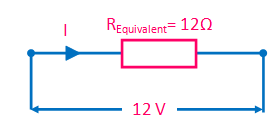Now by using Ohm’s law, the value of the circuit current ( I) is simply calculated as: